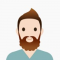## Maximum Sum Decreasing Subsequence in C++Ayush Gupta

Updated on 15-Oct-2020 13:51:08

In this problem, we are given an array arr[] of N integers. Our task is to find the Maximum Sum Decreasing Subsequence in C++.Problem DescriptionWe will find the maximum sum of elements from the array such that the subsequence is strictly decreasing.Let’s take an example to understand the problem, Inputarr[] ... Read More

## Maximum sum from a tree with adjacent levels not allowed in C++Ayush Gupta

Updated on 15-Oct-2020 13:45:55

In this problem, we are given a binary tree consisting of positive numbers. Our task is to create a program to find the Maximum sum from a tree with adjacent levels not allowed in C++.Code DescriptionHere, we will find the maximum sum of node of the tree in such a ... Read More

## Maximum sum from three arrays such that picking elements consecutively from same is not allowed in C++Ayush Gupta

Updated on 15-Oct-2020 12:34:25

In this problem, we are given three arrays arr1[], arr2[], and arr3[] all of size N. Our task is to create a program to find the Maximum sum from three arrays such that picking elements consecutively from same is not allowed in C++.Problem DescriptionWe will find the maximum sum by ... Read More

## Maximum sum in a 2 x n grid such that no two elements are adjacent in C++Ayush Gupta

Updated on 15-Oct-2020 12:32:24

In this problem, we are given a rectangular grid of size 2 x n. Our task is to create a program to find the Maximum sum in a 2 x n grid such that no two elements are adjacent in C++.Problem DescriptionTo find the maximum sum, we cannot select elements ... Read More

## Maximum sum in circular array such that no two elements are adjacent in C++Ayush Gupta

Updated on 15-Oct-2020 12:30:13

In this problem, we are given a circular array cirArr[]. Our task is to create a program to find the Maximum sum in circular array such that no two elements are adjacent in C++.Problem DescriptionFor the circular array, we need to find the maximum sum sum of elements of the ... Read More

## Maximum sum increasing subsequence from a prefix and a given element after prefix is must in C++Ayush Gupta

Updated on 15-Oct-2020 12:26:32

In this problem, we are given an array arr[] of N integers and two index values x and y. Our task is to create a program to find the Maximum sum increasing subsequence from a prefix and a given element after prefix is must in C++.Problem DescriptionWe will find the ... Read More

## Maximum Sum Increasing Subsequence using Binary Indexed Tree in C++Ayush Gupta

Updated on 15-Oct-2020 12:21:46

In this problem, we are given an array arr[] of N elements. Our task is to create a program to find the maximum Sum Increasing Subsequence using Binary Indexed Tree in C++.Let’s take an example to understand the problem, Inputarr[] = {4, 1, 9, 2, 3, 7}Output13ExplanationMaximum increasing subsequence is ... Read More

## Maximum sum of absolute difference of any permutation in C++Ayush Gupta

Updated on 15-Oct-2020 12:19:26

In this problem, we are given an array. Our task is to create a program to find the Maximum sum of absolute difference of any permutation in C++.Problem DescriptionWe will be finding all permutation of the elements of the given array. And then finding the sum of the absolute difference ... Read More

## Maximum sum of difference of adjacent elements in C++Ayush Gupta

Updated on 15-Oct-2020 12:17:54

In this problem, we are given a number N. Our task is to create a program to find the Maximum sum of difference of adjacent elements in C++.Problem DescriptionWe will find the Maximum sum of the absolute difference between the adjacent elements of all permutation arrays.Let’s take an example to ... Read More

## Maximum sum of distinct numbers such that LCM of these numbers is N in C++Ayush Gupta

Updated on 15-Oct-2020 12:16:27

In this problem, we are a number N. Our task is to create a program to find the Maximum sum of distinct numbers such that LCM of these numbers is N in C++.Problem DescriptionWe need to find the sum of all factors of the number N. And add all distinct ... Read More